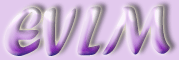Events and the sample space

Target: On completion of this worksheet you should be able to find the probability of simple events and mutually exclusive events. You should also be able to find the probability of independent events and the probability of a combination of events using ‘and’ and ‘or’.

# 1  Introduction

If we toss an ordinary coin the chance of getting a head is 1 out of 2. We say that the probability of getting a head is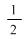. This is because there is only one way of getting a head but two possible outcomes. Each possible outcome of an experiment (in this case either a head or a tail) is called an event. The set of all possible outcomes (in this case the set including heads and tails) is called the sample space.

Similarly the probability of getting a tail is also.

If we consider a six sided dice. Each number has an equal chance of appearing when the dice is thrown.

So the probability of throwing a six is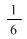.

We write P( throwing a 6 ) =.

In general, P(event)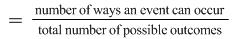So, P(even number)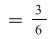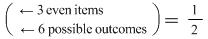.

If we toss a biased coin we do not know what the probability of getting a head is. The only way we can find out is to experiment by tossing the coin many times. The more tosses we do the more accurate will be the estimate of the probability.

If an event cannot occur then the probability is 0.

If an event is certain to occur then the probability is 1.

## 1.1  Examples

A card is picked from a well shuffled pack of 52 playing cards. Find the probability that the card is:

1. A king

Solutions:

1. There are 4 kings in the pack of cards. The probability of a king is therefore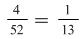.

2. There are 13 spades in the pack of cards. The probability of a spade is therefore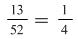## 1.2  Examples

1. A biased dice is thrown 100 times and a 6 appears 40 times.

a) What is the probability of getting a 6

b) What is the probability of not getting a 6?

Solutions:

a) P(6), the probability of getting a 6 is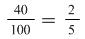.

b) P(not a 6), the probability of not getting a 6 is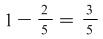.

2. A manufacturer produces bolts of which 2 out of 100 are defective. What is the probability of a bolt not being defective?

P(defective bolt) =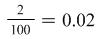.

P(bolt not defective) = 1 – 0.02 = 0.98.

Mutually exclusive events

# 2  Mutually exclusive events

If a dice is thrown then two possible events are ‘getting a 5’ and ‘getting a 6’. If the dice is thrown once then we cannot have both of these events occurring . We say that these events are mutually exclusive. If we consider two different events ‘getting a 3’ and ‘getting an odd number’ then these are not mutually exclusive because if we throw a 3 then both events have occurred.

## 2.1  Examples

1. A dice is thrown. Find the probability of getting a 1 or 6.

Solution:

Since a 1 and a 6 cannot be thrown simultaneously, they are mutually exclusive events. Consequently

P(1 or 6) = P(1) + P(6)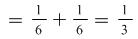.

2. Two students, Ali and Ben, take part in a race. The probability that Ali wins the race is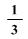and the probability that Ben wins is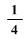. What is the probability that either Ali or Ben wins the race? ( Assume a deadheat is not possible ).

The two events ‘Ali wins the race’ and ‘Ben wins the race’ are mutually exclusive. So P(Ali or Ben wins) = P(Ali wins) + P(Ben wins) =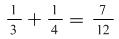.

# 3  Independent events

Suppose a coin is tossed and a dice is thrown. The events ‘get a head’ and ‘get a 4’ are independent events as one event does not affect the other. In this case

P(head and 4)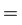P(head)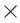P(4)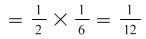.

In general if A and B are two independent events then P(A and B)P(A)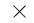P(B).

## 3.1  Examples

1. If a red dice and a blue dice are thrown what is the probability of getting a 2 on the red dice and a 3 on the blue dice?

Solution: These events are independent so P(2 on red and 3 on blue)P(2 on red)P(3 on blue)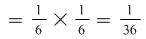.

2. A coin is tossed twice. Find the probability of getting 2 heads.

Solution: Let the probability of getting 2 heads be denoted by P(2 H)P(H on 1st throw)P(H on 2nd throw)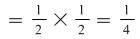.

3. A manufacturer produces components and packs them in boxes of 20. In one particular box it is known that there are 3 defective items. If two components are picked from this box what is the probability that both are defective?

Solution:

P(1st defective)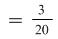.

Now, having picked one item, there are only 19 left in the box and 2 defectives. So

P(2nd defective)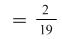.

P(both defective)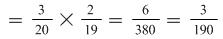.

4. A bag contains 5 red sweets and 7 blue sweets. Find the probability that if two sweets are picked they are not both red.

P(2 red)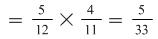.

P(not both red)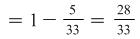.

5. A company manufactures CDs of which 3% are faulty. What is the probability of getting exactly one faulty CD in a box of 3?

P(faulty CD) = 0.03 P( good CD)

P(faulty, good, good)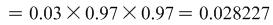But the order could be good, faulty, good or good, good, faulty. In each of these the probability is the same as above. These are mutually exclusive events so we can add the probabilities ie multiply by 3

P(1 faulty CD in box of 3)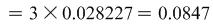to 3 sig fig.

6. A bag contains 5 red balls and 7 green balls. Four balls are picked out of the bag. What is the probability that two are red and two are green?

P(R,R,G,G)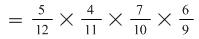.

The denominator decreases by 1 each time a ball is taken out. We must find the probability for all the different ways of getting 2 red and 2 green and then add these together.

P(R,G,R,G)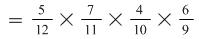.

Notice that we have the same numerators but in a different order (the denominators are exactly the same). This will be the same for all cases so we must find how many ways there are of picking 2 red and 2 green balls:

RRGG, RGRG, RGGR, GGRR, GRGR, GRRG. There are 6 different ways so

P(2R &2G)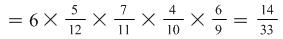.

Notation: In the above we take account of what has already happened. We say ‘probability of a red given that a red has already been picked’ and write P(red | red already picked).

In general if A and B are two events then P(B|A) means probability of B occurring given that A has already occurred. Also

P(A and B) = P(A)P(B|A).

Suppose we draw 1 card from a well shuffled pack of 52 playing cards. Consider the 2 events ‘an ace is drawn’ and ‘a club is drawn’. We want to find

P(an ace or a club). We can list the possible cards for each event:

 Event 1 Event 2 Ace of ♦, Ace of ♠,Ace of ♦, Ace of ♠ Ace of ♣, 2 ♣,3 ♣, 4 ♣, …,queen ♣, king ♣

The Ace of ♣ is in both columns so the events are not mutually exclusive. Now

P(ace)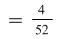, P(♣)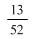, P(ace ♣)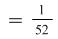.

P(an ace or a club)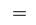P(ace)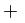P(♣)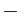P(ace ♣)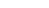Includes ace ♣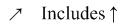ace ♣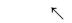Takes one of the ace ♣ away

P(ace or club)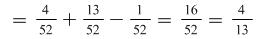.

In general given 2 events A and B

P(A or B) = P(A) + P(B) – P(A and B)